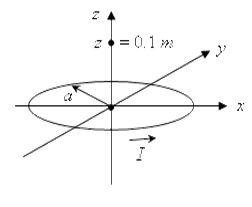# Circular loop magnetic field

## Homework Statement

As shown in the figure below, a circular loop of radius a = 0.1 m lies in the horizontal x-y plane with its center located at the origin, with steady current I = 2.0 A circulating in a counter-clockwise direction in the loop. The magnetic field strength B(0,0,z) at the observation point (x, y, z) = (0, 0, 0.1 m) and its direction, due to the current I flowing in the circular loop is:
(a) B(0,0,z=0.1m) = 0.628 × 10-7 T, -z direction
(b) B(0,0,z=0.1m) = 2.323 × 10-6 T, +z direction
(c) B(0,0,z=0.1m) = 3.678 × 10-6 T, -z direction
(d) B(0,0,z=0.1m) = 4.443 × 10-6 T, +z direction

http://i.imgur.com/i6Zxf.pngAmpere's Law

## The Attempt at a Solution

I tried Ampere's law, but I am not sure what is B dot dl (especially angle) and what is the path in this case...

Thx

dynamicsolo
Homework Helper
I believe you want the Biot-Savart Law, since we are dealing with a current loop, rather than a current-carrying straight wire.

At any point on the loop, which way does the magnetic field circulate? At the observation point, what is going to happen to the "horizontal components" (the parts parallel to the xy-plane) of all the bits of magnetic field coming from the loop? What will that mean as far as how the bits of magnetic field add up at ( 0, 0, 0.1 m.)?

I believe you want the Biot-Savart Law, since we are dealing with a current loop, rather than a current-carrying straight wire.

At any point on the loop, which way does the magnetic field circulate? At the observation point, what is going to happen to the "horizontal components" (the parts parallel to the xy-plane) of all the bits of magnetic field coming from the loop? What will that mean as far as how the bits of magnetic field add up at ( 0, 0, 0.1 m.)?

At any point on the loop, which way does the magnetic field circulate?

Up, by RHR

At the observation point, what is going to happen to the "horizontal components" (the parts parallel to the xy-plane) of all the bits of magnetic field coming from the loop?

Bcos(45)?

What will that mean as far as how the bits of magnetic field add up at ( 0, 0, 0.1 m.)?

integral Bcos(45)dl?

ehild
Homework Helper
Thanks. But there is an angle in this case....

If you scroll down the page you arrive at the title "Magnetic field on the axis".

ehild

If you scroll down the page you arrive at the title "Magnetic field on the axis".

ehild

Okay. Thanks